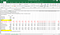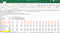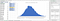# DI: Estimation of IRR and Monte Carlo Simulation of NPV by Excel

Most of the DCF (Discounted Cash Flow) model is based on a deterministic assumption that all growth rates and discount rates are known apriori, by means of this deterministic DCF model, we can then estimate the Internal Rate of Return (IRR), i.e. the discount rate such that Net Present Value, NPV = 0.

1. Deterministic DCF Model and Estimation of IRR

(If you are familiar with this deterministic DCF model, you may skip this section and jump to the Monte Carlo Simulation section.)

However, IRR cannot be solved by a mathematical formula, it can only be obtained by a trial-and-error approach. Fortunately, Excel provides such a trial-and-error function, i.e. Goal-seek. Figure 1 shows a DCF example of a shopping mall, assuming the growth rate and the inflation rate are fixed at 2% and 1% respectively, and the lease is for 40 years, then the NPV will be dependent on the discount rate. We can make use of the Top Bar Command: Data -> What if -> Goal Seek, and set IRR as the variable, and the NPV =0 as the goal. It will then estimate the IRR such that NPV=0, if it is estimable.Figure 1 Estimation of IRR for a DCF Model by Excel Goal Seek Function

2. Monte Carlo Simulations — Panel A (Normal Distribution)

In real life, we seldom know future growth rates and inflation rates apriori. Yet, based on past data, we probably can tell its past average and standard deviation. Thus, a DCF should not be a deterministic model, but a stochastic one.

Figure 2 shows Panel A of estimating the growth rates and inflation rates based on a randomized function of an assumed Normal Distribution. The Excel function is NORMINV(RAND(), Ave, SD). Then the estimated NPV is not a fixed value but will fluctuate in every scenario. Figure 2 is just one of the examples.Figure 2 Randomized Growth Rates and Inflation Rates based on an assumed Normal Distribution

3. Monte Carlo Simulations — Panel B (What is the Prob of NPV < 0?)

Since the estimated NPV fluctuates, it is reasonable to ask the distribution pattern of the estimated NPVs if we can simulate the estimations tens of thousand times. Excel also provides a very helpful function (Data -> What if -> Data Table) for repeating the simulations numerous times with each row showing each simulated NPV, as shown in Figure 3.

For investors, they may be interested in knowing the chance of making a loss, i.e. NPV < 0. After repeating the simulations 10,000 times, we can count how many times that NPV < 0, and report the probability of making a loss. Excel provides a COUNTIF(data range, “<0”) function. Since the simulations vary, Figure 3 is only an example.

4. Overall Distribution Pattern of the Simulated NPVs

If we want to plot the overall distribution pattern of the simulated NPVs, we can make use of the Pivot Table function of Excel. Inserting a Pivot Table of the data range of the simulated NPVs will make the chart easily. Figure 4 shows an example, drag the data into the ROW and VALUE boxes in the right-hand corner, and right-click any one of the data will show a pull-down menu, click GROUP will allow you to choose a Start and an End Values, and the Interval width for constructing a histogram. Top bar command also provides ANALYSE to draw a Pivot Chart. The distribution can then be drawn directly.Figure 4 Use the Pivot Table function of Excel to Plot the Distribution of the Simulated NPVs

Monte Carlo Simulation is a very powerful tool for data analysis, which can provide insights into real-life problems. In the past, when computer capacity is limited, we resort to deterministic DCF models for simple calculation reasons. Nowadays, when even Excel can perform Monte Carlo Simulations, there is no excuse to stick to deterministic DCF models, as it would ignore the risk of fluctuating changes in the future. It is time to change the teaching materials and empower our students to analyze data both deterministically and stochastically.

For reference, you may also refer to Luis Qlano’s (2010) Youtube that shows a similar Monte Carlo Simulation — NPV example,

Written by